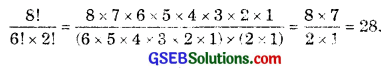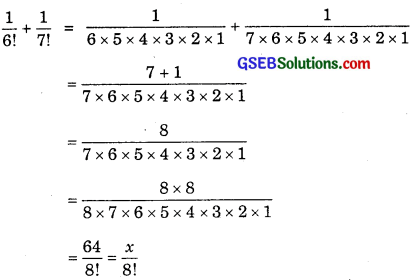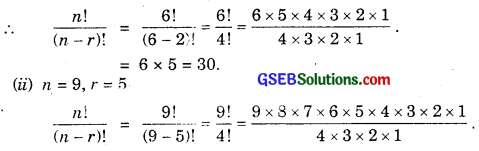# GSEB Solutions Class 11 Maths Chapter 7 Permutations and Combinations Ex 7.2

Gujarat Board GSEB Textbook Solutions Class 11 Maths Chapter 7 Permutations and Combinations Ex 7.2 Textbook Questions and Answers.

## Gujarat Board Textbook Solutions Class 11 Maths Chapter 7 Permutations and Combinations Ex 7.2

Question 1.
Evaluate:

1. 8!
2. 4! – 3!

Solution:
1. 8! = 8 × 7 × 6 × 5 × 4 × 3 × 2 × 1
= 40320.

2. 4! – 3! = 4 × 3 × 2 × 1 – 3 × 2 × 1
= 24 – 6
= 18.Question 2.
Is 3! + 4! = 7!?
Solution:
3! = 3 × 2 × 1 = 6.
4! = 4 × 3 × 2 × 1 = 24.
So, L.H.S. = 3! + 4!
= 6 + 24
= 30.
Now, R.H.S. = 7! = 7 × 6 × 5 × 4 × 3 × 2 × 1
= 5040 ≠ L.H.S.
Hence, 3! + 4! ≠ 7!.Question 3.
Compute $$\frac{8!}{6!×2!}$$.
Solution:Question 4.
If $$\frac{1}{6!}$$ + $$\frac{1}{7!}$$ = $$\frac{x}{8!}$$, find x?
Solution:∴ x = 64.Question 5.
Evaluate $$\frac{n!}{n – r}$$, when
(i) n = 6, r = 2
(ii) n = 9, r = 5
Solution:
(i) n = 6, r = 2∴ 9 × 8 : 7 × 6 × 5
= 15120.